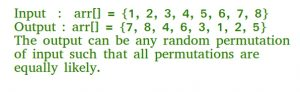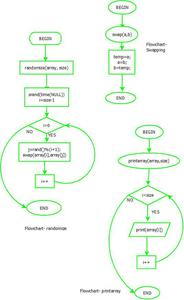Open In App
Related Articles

# Shuffle a given array using Fisher–Yates shuffle Algorithm

Given an array, write a program to generate a random permutation of array elements. This question is also asked as “shuffle a deck of cards” or “randomize a given array”. Here shuffle means that every permutation of array element should be equally likely.Let the given array be arr[]. A simple solution is to create an auxiliary array temp[] which is initially a copy of arr[]. Randomly select an element from temp[], copy the randomly selected element to arr, and remove the selected element from temp[]. Repeat the same process n times and keep copying elements to arr, arr, … . The time complexity of this solution will be O(n^2).

Fisher–Yates shuffle Algorithm works in O(n) time complexity. The assumption here is, we are given a function rand() that generates a random number in O(1) time. The idea is to start from the last element and swap it with a randomly selected element from the whole array (including the last). Now consider the array from 0 to n-2 (size reduced by 1), and repeat the process till we hit the first element.

Following is the detailed algorithm that is as follows:

```To shuffle an array a of n elements (indices 0..n-1):
for i from n - 1 downto 1 do
j = random integer with 0 <= j <= i
exchange a[j] and a[i]```

Flowchart:flowchart

Following is an implementation of this algorithm.

## C++

 `// C++ Program to shuffle a given array``#include``#include ``#include ``using` `namespace` `std;` `// A utility function to swap to integers``void` `swap (``int` `*a, ``int` `*b)``{``    ``int` `temp = *a;``    ``*a = *b;``    ``*b = temp;``}` `// A utility function to print an array``void` `printArray (``int` `arr[], ``int` `n)``{``    ``for` `(``int` `i = 0; i < n; i++)``        ``cout << arr[i] << ``" "``;``    ``cout << ``"\n"``;``}` `// A function to generate a random``// permutation of arr[]``void` `randomize (``int` `arr[], ``int` `n)``{``    ``// Use a different seed value so that``    ``// we don't get same result each time``    ``// we run this program``    ``srand` `(``time``(NULL));` `    ``// Start from the last element and swap``    ``// one by one. We don't need to run for``    ``// the first element that's why i > 0``    ``for` `(``int` `i = n - 1; i > 0; i--)``    ``{``        ``// Pick a random index from 0 to i``        ``int` `j = ``rand``() % (i + 1);` `        ``// Swap arr[i] with the element``        ``// at random index``        ``swap(&arr[i], &arr[j]);``    ``}``}` `// Driver Code``int` `main()``{``    ``int` `arr[] = {1, 2, 3, 4, 5, 6, 7, 8};``    ``int` `n = ``sizeof``(arr) / ``sizeof``(arr);``    ``randomize (arr, n);``    ``printArray(arr, n);` `    ``return` `0;``}` `// This code is contributed by``// rathbhupendra`

## C

 `// C Program to shuffle a given array` `#include ``#include ``#include ` `// A utility function to swap to integers``void` `swap (``int` `*a, ``int` `*b)``{``    ``int` `temp = *a;``    ``*a = *b;``    ``*b = temp;``}` `// A utility function to print an array``void` `printArray (``int` `arr[], ``int` `n)``{``    ``for` `(``int` `i = 0; i < n; i++)``        ``printf``(``"%d "``, arr[i]);``    ``printf``(``"\n"``);``}` `// A function to generate a random permutation of arr[]``void` `randomize ( ``int` `arr[], ``int` `n )``{``    ``// Use a different seed value so that we don't get same``    ``// result each time we run this program``    ``srand` `( ``time``(NULL) );` `    ``// Start from the last element and swap one by one. We don't``    ``// need to run for the first element that's why i > 0``    ``for` `(``int` `i = n-1; i > 0; i--)``    ``{``        ``// Pick a random index from 0 to i``        ``int` `j = ``rand``() % (i+1);` `        ``// Swap arr[i] with the element at random index``        ``swap(&arr[i], &arr[j]);``    ``}``}` `// Driver program to test above function.``int` `main()``{``    ``int` `arr[] = {1, 2, 3, 4, 5, 6, 7, 8};``    ``int` `n = ``sizeof``(arr)/ ``sizeof``(arr);``    ``randomize (arr, n);``    ``printArray(arr, n);` `    ``return` `0;``}`

## Java

 `// Java Program to shuffle a given array``import` `java.util.Random;``import` `java.util.Arrays;``public` `class` `ShuffleRand``{``    ``// A Function to generate a random permutation of arr[]``    ``static` `void` `randomize( ``int` `arr[], ``int` `n)``    ``{``        ``// Creating a object for Random class``        ``Random r = ``new` `Random();``        ` `        ``// Start from the last element and swap one by one. We don't``        ``// need to run for the first element that's why i > 0``        ``for` `(``int` `i = n-``1``; i > ``0``; i--) {``            ` `            ``// Pick a random index from 0 to i``            ``int` `j = r.nextInt(i+``1``);``            ` `            ``// Swap arr[i] with the element at random index``            ``int` `temp = arr[i];``            ``arr[i] = arr[j];``            ``arr[j] = temp;``        ``}``        ``// Prints the random array``        ``System.out.println(Arrays.toString(arr));``    ``}``    ` `    ``// Driver Program to test above function``    ``public` `static` `void` `main(String[] args)``    ``{``        ` `         ``int``[] arr = {``1``, ``2``, ``3``, ``4``, ``5``, ``6``, ``7``, ``8``};``         ``int` `n = arr.length;``         ``randomize (arr, n);``    ``}``}``// This code is contributed by Sumit Ghosh`

## Python3

 `# Python Program to shuffle a given array``from` `random ``import` `randint` `# A function to generate a random permutation of arr[]``def` `randomize (arr, n):``    ``# Start from the last element and swap one by one. We don't``    ``# need to run for the first element that's why i > 0``    ``for` `i ``in` `range``(n``-``1``,``0``,``-``1``):``        ``# Pick a random index from 0 to i``        ``j ``=` `randint(``0``,i``+``1``)` `        ``# Swap arr[i] with the element at random index``        ``arr[i],arr[j] ``=` `arr[j],arr[i]``    ``return` `arr` `# Driver program to test above function.``arr ``=` `[``1``, ``2``, ``3``, ``4``, ``5``, ``6``, ``7``, ``8``]``n ``=` `len``(arr)``print``(randomize(arr, n))`  `# This code is contributed by Pratik Chhajer`

## C#

 `// C# Code for Number of digits``// in the product of two numbers``using` `System;` `class` `GFG``{``// A Function to generate a``// random permutation of arr[]``    ``static` `void` `randomize(``int` `[]arr, ``int` `n)``    ``{``        ``// Creating a object``        ``// for Random class``        ``Random r = ``new` `Random();``        ` `        ``// Start from the last element and``        ``// swap one by one. We don't need to``        ``// run for the first element``        ``// that's why i > 0``        ``for` `(``int` `i = n - 1; i > 0; i--)``        ``{``            ` `            ``// Pick a random index``            ``// from 0 to i``            ``int` `j = r.Next(0, i+1);``            ` `            ``// Swap arr[i] with the``            ``// element at random index``            ``int` `temp = arr[i];``            ``arr[i] = arr[j];``            ``arr[j] = temp;``        ``}``        ``// Prints the random array``        ``for` `(``int` `i = 0; i < n; i++)``        ``Console.Write(arr[i] + ``" "``);``    ``}``    ` `    ` `// Driver Code``static` `void` `Main()``{``    ``int``[] arr = {1, 2, 3, 4,``                 ``5, 6, 7, 8};``    ``int` `n = arr.Length;``    ``randomize (arr, n);``}``}` `// This code is contributed by Sam007`

## PHP

 ` 0``    ``for``(``\$i` `= ``\$n` `- 1; ``\$i` `>= 0; ``\$i``--)``    ``{``        ``// Pick a random index``        ``// from 0 to i``        ``\$j` `= rand(0, ``\$i``+1);` `        ``// Swap arr[i] with the``        ``// element at random index``        ``\$tmp` `= ``\$arr``[``\$i``];``        ``\$arr``[``\$i``] = ``\$arr``[``\$j``];``        ``\$arr``[``\$j``] = ``\$tmp``;``    ``}``    ``for``(``\$i` `= 0; ``\$i` `< ``\$n``; ``\$i``++)``    ``echo` `\$arr``[``\$i``].``" "``;``}` `// Driver Code``\$arr` `= ``array``(1, 2, 3, 4,``             ``5, 6, 7, 8);``\$n` `= ``count``(``\$arr``);``randomize(``\$arr``, ``\$n``);` `// This code is contributed by mits``?>`

## Javascript

 `// JavaScript Program to shuffle a given array` `// A function to print an array``function` `printArray (arr)``{``    ``let ans = ``''``;``    ``for` `(let i = 0; i < arr.length; i++)``    ``{``        ``ans += arr[i] + ``" "``;``    ``}``    ``console.log(ans);``}` `// A function to generate a random``// permutation of arr``function` `randomize (arr)``{` `    ``// Start from the last element and swap``    ``// one by one. We don't need to run for``    ``// the first element that's why i > 0``    ``for` `(let i = arr.length - 1; i > 0; i--)``    ``{``    ` `        ``// Pick a random index from 0 to i inclusive``        ``let j = Math.floor(Math.random() * (i + 1));` `        ``// Swap arr[i] with the element``        ``// at random index``        ``[arr[i], arr[j]] = [arr[j], arr[i]];``    ``}``}` `// Driver Code``let arr = [1, 2, 3, 4, 5, 6, 7, 8];``randomize (arr);``printArray(arr);` `// This code is contributed by rohitsingh07052.`

Output :

`7 8 4 6 3 1 2 5`

The above function assumes that rand() generates a random number.

Time Complexity: O(n), assuming that the function rand() takes O(1) time., Auxiliary Space: O(1)

How does this work?

The probability that the ith element (including the last one) goes to the last position is 1/n, because we randomly pick an element in the first iteration. This means that each element has an equal chance of ending up in the last position.

The probability that the ith element goes to the second-to-last position can be proven to be 1/n by dividing it into two cases:

Case 1: i = n-1 (index of last element):
In this case, the probability of the last element going to the second-to-last position is equal to the probability that the last element does not stay at its original position, multiplied by the probability that the index picked in the previous step is picked again so that the last element is swapped.

This means that the probability is: ((n-1)/n) x (1/(n-1)) = 1/n

Case 2: 0 < i < n-1 (index of non-last element):
In this case, the probability of the ith element going to the second-to-last position is equal to the probability that the ith element is not picked in the previous iteration, multiplied by the probability that the ith element is picked in this iteration.

This means that the probability is: ((n-1)/n) x (1/(n-1)) = 1/n

We can easily generalize the proof for any other position by applying the same logic. For example, the probability that the ith element goes to the third-to-last position is 1/n, because it is equally likely to be picked in any iteration.
For example, if we have an array with 5 elements, each element has a 1/5 chance of ending up in the last position. And if we shuffle the array multiple times, we should see that each element ends up in the last position about 1/5 of the time on average.

This also applies to other positions in the array. For example, each element has a 1/5 chance of ending up in the second-to-last position, because it is equally likely to be picked in any iteration.

Overall, this means that the shuffle is random and fair, because each element has an equal chance of ending up in any position.